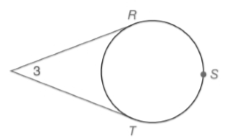Chapter 6.2, Problem 13EElementary Geometry For College St...

7th Edition
Alexander + 2 others
ISBN: 9781337614085

Solutions

Chapter
SectionElementary Geometry For College St...

7th Edition
Alexander + 2 others
ISBN: 9781337614085
Textbook Problem

Given: R S ⌢ ≅ S T ⌢ ≅ R T ⌢ Find: a) m R T ⌢ b) m R S T ⌢ c) m ∠ 3Exercises 12 ,   13

To determine

a)

To find:Explanation

Given,

As the values are congruent, all the values are equal.

mRS=ST=RT=x

To determine

b)

To find:

mRST

To determine

c)

To find:

m3

Still sussing out bartleby?

Check out a sample textbook solution.

See a sample solution

The Solution to Your Study Problems

Bartleby provides explanations to thousands of textbook problems written by our experts, many with advanced degrees!

Get Started

In Exercises 1-6, simplify the expression. 3. 12t2+12t+34t21

Applied Calculus for the Managerial, Life, and Social Sciences: A Brief Approach

Change 64 ft/s to mi/h.

Elementary Technical Mathematics

If f(x) = x + 4 and h(x) = 4x 1, find a function g such that g f = h.

Single Variable Calculus: Early Transcendentals, Volume I

In Exercises 3-11, find the derivative of the function. f(x)=12x

Calculus: An Applied Approach (MindTap Course List)

Sketch the graphs of the equations in Exercises 512. xy=x2+1

Finite Mathematics and Applied Calculus (MindTap Course List)

Calculate y'. 23. y = (1 x1)1

Single Variable Calculus: Early Transcendentals

The solution to xdydx2y=x3 is: a) y=ex3+Cx2 b) y=ex3+x2+C c) y=x3+x2+C d) y=x3+Cx2

Study Guide for Stewart's Single Variable Calculus: Early Transcendentals, 8th

(i · j)k = k 2-k 0 (the number 0) 0 (the zero vector)

Study Guide for Stewart's Multivariable Calculus, 8th